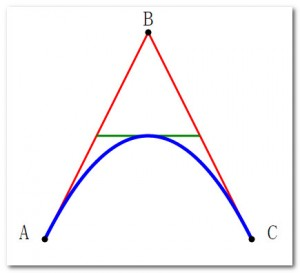A-A+

# D3.js学习笔记一:认识SVG图形

2015年02月11日 阅读 1,458 views 次

<svg> <line x1="0" y1="0" x2="100" y2="100" stroke="#F00"/> </svg>

<svg> <rect x="0" y="0" width="100" height="100"/> </svg>

SVG形状标签支持CSS样式，使用style来为它定义样式，例如：用以下代码可以画一个100×100的带有黄色边框的红色透明圆角矩形,为显示透明度，在底层加了一个黑色背景框。

<svg> <rect x="0" y="0" width="100" height="100" style="fill:#000;"/> <rect x="50" y="50" width="100" height="100" rx="10" ry="10" style="fill:#F00;stroke-width:1;stroke:#FF0;opacity:0.9;"/> </svg>

CSS样式说明

• rx 和 ry 属性可使矩形产生圆角。
• CSS 的 fill 属性定义矩形的填充颜色（rgb 值、颜色名或者十六进制值）
• CSS 的 stroke-width 属性定义矩形边框的宽度
• CSS 的 stroke 属性定义矩形边框的颜色
• CSS 的 fill-opacity 属性定义填充颜色透明度（合法的范围是：0 - 1）
• CSS 的 stroke-opacity 属性定义笔触颜色的透明度（合法的范围是：0 - 1）

<svg> <circle cx="100" cy="100" r="50"/> </svg>

<svg> <ellipse cx="100" cy="100" rx="100" ry="100" style="fill:#F00;stroke-width:1;stroke:#FF0;opacity:0.9;"/> <ellipse cx="100" cy="100" rx="100" ry="50"/> </svg>

<svg> <polygon points="0,0 100,0 100,100 0,100" style="fill:#F00;stroke-width:1;stroke:#FF0;opacity:0.5;"/> <polygon points="50,0 100,50 0,50" style="fill:#FF0;stroke-width:1;stroke:#FF0;opacity:0.5;"/> </svg>

<svg> <polyline points="0,5 100,20 200,65 300,48 400,37" style="fill:#FFF;stroke:#F00;stroke-width:2"/> </svg>

• M = moveto 将画笔移动到
• L = lineto 从画笔处到此点画线
• H = horizontal lineto 从画笔处到此点画线
• V = vertical lineto 从画笔处到此点画线
• C = curveto 从画笔处到此点画曲线
• S = smooth curveto 从画笔处到此点画平滑线
• Q = quadratic Belzier curve 从画笔处到此点画贝塞尔线
• T = smooth quadratic Belzier curveto 从画笔处到此点画贝塞尔线
• A = elliptical Arc
• Z = closepath 结束描绘

<svg xmlns="http://www.w3.org/2000/svg" version="1.1">
<path id="lineAB" d="M 100 350 l 150 -300" stroke="red"
stroke-width="3" fill="none" />
<path id="lineBC" d="M 250 50 l 150 300" stroke="red"
stroke-width="3" fill="none" />
<path d="M 175 200 l 150 0" stroke="green" stroke-width="3"
fill="none" />
<path d="M 100 350 q 150 -300 300 0" stroke="blue"
stroke-width="5" fill="none" />
<!-- Mark relevant points -->
<g stroke="black" stroke-width="3" fill="black">
<circle id="pointA" cx="100" cy="350" r="3" />
<circle id="pointB" cx="250" cy="50" r="3" />
<circle id="pointC" cx="400" cy="350" r="3" />
</g>
<!-- Label the points -->
<g font-size="30" font="sans-serif" fill="black" stroke="none"
text-anchor="middle">
<text x="100" y="350" dx="-30">A</text>
<text x="250" y="50" dy="-10">B</text>
<text x="400" y="350" dx="30">C</text>
</g>
</svg>[微信] 扫描二维码打赏[支付宝] 扫描二维码打赏